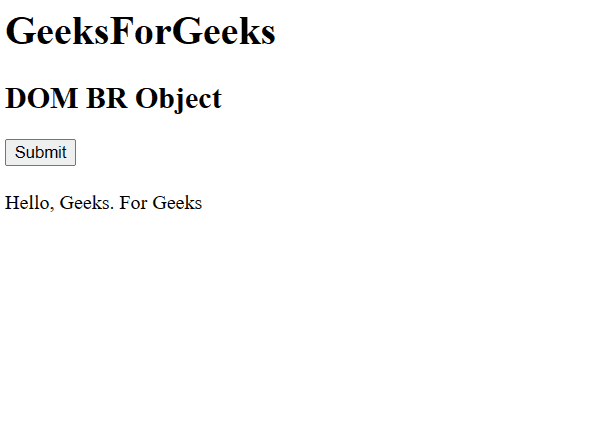# HTML DOM BR Object

The DOM BR Object is used to represent the HTML <br> element. The br element is accessed by getElementById().

Syntax:

`document.getElementById(id)`

Where “id” is the ID assigned to the br tag.

Property:

• clear: It is used to Sets or return the flow of text around floating objects

Example 1: In this example, we will use DOM BR Object

## HTML

 `` `<``html``>` `  `  `<``head``>` `    ``<``title``>HTML | DOM BR Object` `    ``<``style``>` `        ``body {` `            ``text-align: center;` `        ``}` `        ``h1 {` `            ``color: green;` `        ``}` `    ``` `` `  `  `<``body``>` `    ``<``h1``>GeeksforGeeks` `    ``<``h2``>DOM br Object` `    ``` `    ``<``p``>` `          ``GeeksforGeeks:` `        ``<``br` `id``=``"GFG"``> Computer science portal` `    ``` `    ``<``button` `onclick``=``"myGeeks()"``>Subnit` `  `  `    ``<``script``>` `        ``function myGeeks() {` `            ``let w = document.getElementById("GFG");` `            ``w.style.display = "none";` `        ``}` `    ``` `` `  `  ``

Output:Example 2: Br Object can be created by using the document.createElement Method.

## HTML

 `` `<``html``>`   `<``body``>` `    ``<``h1``>GeeksForGeeks` `    ``<``h2``>DOM BR Object` `    ``<``button` `onclick``=``"Geeks()"` `            ``style``=``"margin-bottom:20px;"``>` `        ``Submit` `    ``` `    ``<``div` `id``=``"GFG"``>` `        ``<``span``>Hello, ` `        ``<``span``>Geeks.` `        ``<``span``>For` `        ``<``span``>Geeks` `    ``` `  `  `    ``<``script``>` `        ``function Geeks() {` `            ``// Get the div element with id="GFG" ` `            ``let g = document.getElementById("GFG");`   `            ``// Get all span elements inside of div. ` `            ``let f = g.getElementsByTagName("SPAN");`   `            ``//  Create a loop which will insert a br` `            ``//     element before each span element in div,` `            ``//     starting from the second span element.             ` `            ``let j;` `            ``for (j = 1; j < ``f.length``; j++) {` `                ``let ``w` `= ``document``.createElement("BR");` `                ``g.insertBefore(w, f[j]);` `            ``}` `        ``}` `    ``` ``   ``

Output:Supported Browsers: The browser supported by DOM Br Object are listed below: